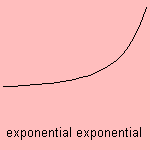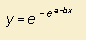# exponential exponential

## exponential

last updated: 2003-07-19The curve is the exponential of an exponential.Its inverse is the logarithmic logarithm, e.g. y = ln (ln x).
This logarithmic logarithm is an approximation of the inverse prime summation.

The Gompertz curve 1) is a linear form of the exponential exponential.

1) the Gompertz relation has the form of: